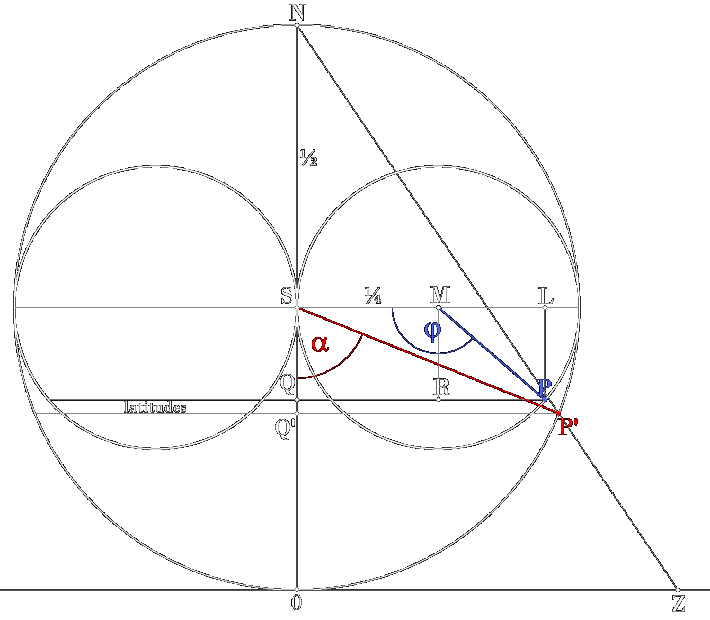conformal mapping from (Riemann) sphere to horn torus surface    endeavour: transfer point P' on sphere to a point P on torus, equivalent angle α → angle φ  (see sketch), without changing shape and angles of projected or mapped figures, e.g. circles    denotation of points: (all points lie on the same plane that contains the main symmetry axis of the horn torus) P' : any point on (any!) sphere, here we particularly cover the Riemann sphere P : mapped point on nested horn torus, but compilation will also be valid for any horn torus! Z : position of related complex number (x,y) on complex plane in case of Riemann sphere mapping 0 : center of complex plane (Z = (0,0)) and south pole of Riemann sphere (plane is tangent to sphere) N : north pole of sphere, origin of Riemannian stereographic projection Z ↔ P' S : common center of sphere and horn torus (not the south pole of sphere, notabene! I insist   on this denotation for the horn torus, because S stands for for Symmetry and Singularity!) M : center of torus bulge cross-section circle Q : intersection of perpendicular from point P on line ON Q' : intersection of perpendicular from point P' on line ON L : auxiliary point on line through S and M, intersection of perpendicular from P R : auxiliary point on line QP, intersection of perpendicular from M    angles: α = ∠0SP'  (0 < α < π, α = 0 for P' = point 0, α = π for P' = N) φ = ∠SMP  (0 < φ < 2π, φ = 0 for P = S  and/or  φ = 2π for P = S) ω : rotation angle around symmetry axis (0N) = position of longitude (meridian) on sphere resp. torus    procedure: we don't declare any appropriate stereographic projection to proof afterwards the conformality but use instead the conditions of conformality to compile and establish the wanted mapping analytically: for this we construct small circles on the surfaces of sphere resp. horn torus around points P' resp. P by equalling their radii that lie on longitudes and on latitudes of sphere and of horn torus respectively. in the sketch the longitudes are the cross-section circles on wich points P' and P are positioned and the latitudes are circles perpendicular to the drawing plane through P' and P with radius Q'P' resp. QP, they lie, half each, in front and behind the drawing plane, Q' and Q being centers of the latitude circles    compilation: for Riemann sphere is valid: length of longitude (meridian) m = 2π·r = 2π·½ = π and length of latitude l = 2π·Q'P' = 2π·½·sinα = π·sinα for horn torus we have as length of longitude m = 2π·¼ = π/2, the latitude is slightly trickier: its radius is QP = QR + RP = SM + ML = ¼·(1 + cos(π - φ)), so latitude l = 2π·QP = ½·π·(1 - cosφ) now we consider infinitesimal circles around P' and P on the surfaces of sphere resp. horn torus and equal their radii dm on longitude and dl on latitude, noting that dω is identic for both figures on sphere is dm = ½·dα, dl = ½·sinα·dω, and so for dm = dl we get  dω = dα / sinα on horn torus is dm = ¼·dφ, dl = ¼·(1 - cosφ)·dω, for dm = dl we get  dω = dφ / (1 - cosφ) finally we have to integrate a relative simple differential equation:  dα / sinα = dφ / (1 - cosφ)homeRiemann sphere:  0N = 1 ,  ∠0NP' = α/2 ,  ∠0ZN = π/2 - α/2 ,  |z| = 0Z   gifnote: the solution is valid for any sphere and any horn torus !  ( → as  pdf )see : numerical computation and check for conformality (Excel-files .xlsx)

solution:(1/sinα)dα  =  (1/(1 - cosφ))dφ    ln(|tan(α/2)|)  =  - cot(φ/2) + C    α  =  2·arctan(e^(-cot(φ/2) + C))    φ  =  2·arccot(-ln(|tan(α/2)|) - C)   (C any real number) continue